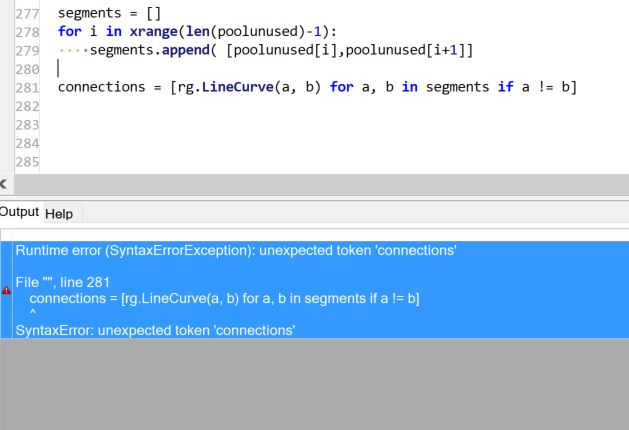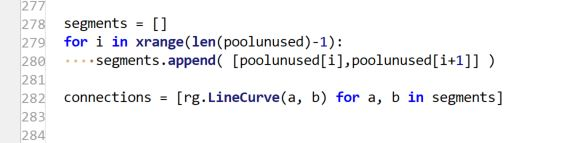# Python Closest Point in Pointgrid, How to use resulting point again for Closest Point Loop

still the same problem

Like this?

``````poolunused = sum_attractors[:]
shortestpathfromattractors = []

counter = 0
while len(poolunused) > 0:
for pt in poolunused:
if counter == 0: start_point0 =  start_point0
else: start_point0 = shortestpathfromattractors[len(shortestpathfromattractors)-1]
ClosestPt = rc.Collections.Point3dList.ClosestIndexInList(poolunused, start_point0)
shortestpathfromattractors.append(poolunused[ClosestPt])
poolunused.pop(ClosestPt)
counter +=1``````

Hi @STUDENT_F,

You can solve this with a recursive function!

Your start point is automatically added to the path points as long as it is part of the Points that you input!

consecutive_closest_points_v1.gh (9.5 KB)

2 Likes

That is a well organized script that works brilliantly for me. Thanks a lot for your support and time!

Do you also know how to get start and end point of each pair?

Sure, simply make the following changes to the script:

``````import Rhino.Geometry as rg

def find_closest_points(start_point, point_cloud, max_recs=float("inf"), _depth=0, _path=[]):
"""Recursively searches a point cloud for consecutive closest points.

Args:
test_point: A point to search from.
point_cloud: A point cloud to search.
max_recs: An optional maximum number of recursions.
_depth: A current recursion depth.
_path: A current nested list of point pairs.

Returns:
The nested list of consecutive point pairs.
"""
if point_cloud.Count > 0:
pt_cloud = point_cloud.Duplicate()
closest_idx = pt_cloud.ClosestPoint(start_point)
closest_pt = rg.Point3d(pt_cloud[closest_idx].X,
pt_cloud[closest_idx].Y,
pt_cloud[closest_idx].Z)
_path.append([start_point, closest_pt]) # Change this...
pt_cloud.RemoveAt(closest_idx)
if point_cloud.Count > 0 and _depth < max_recs:
_depth +=1
return find_closest_points(closest_pt, pt_cloud, max_recs, _depth, _path)
return _path

# Get the start point
start_pt = Points[iStartPoint]
# Create the point cloud
attractor_pts = rg.PointCloud(Points)

# Get the consecutive point pairs
pairs = find_closest_points(start_pt, attractor_pts) # ... and this
print pairs

# Flatten the point pairs list (for the polyline construction)
path = [pair for pair in pairs] # ... and this

# Outputs
a = path # ... and finally this
``````

When flattening the list of point pairs, you may wander why we simply keep the second item from each pair. Well, it has to do with the start point! If the start point is a member of the attractor points, it finds itself as the closest point at the very first recursion.
In the first script, this was great, since the first point to append to the path list was the start point anyway.
However, in pairs script, at the very first recursion, the start point finds itself as its closest point, and thus forms a pair with itself. At the second recursion, it looks for another closest point, but this time, its doppelgänger was already deleted from the point cloud, so it finds another point this time.

Example:
Pairs list:
`[[start_point, start_point], [start_point, other_point0], [other_point0, other_point1], … [other_pointX, other_pointY]]`
Flat list:
`[start_point, other_point0, other_point1, … , other_pointX, other_pointY]`

Furthermore, you can also limit the length of the path, by limiting the maximum recursion depth that is currently set to infinity. The maximum recursion depth is mainly a security parameter that you can set to prevent huge numbers of recursions though.

Ok Python version:)

``````def infinity():
i = 0
while True:
yield i
i+=1

for i in infinity():
if i == 1000000:
print('done')
break
``````

A rare example of Python being much more verbose than C

Thank you. Unfortunatly when I print pairs, there are no pairs. I need branches with two elements. Start and Endpoint.
So at the end: listofpairs = [[a,b], [b,c], [c,d], …]

When printing from the GHPython component, there should be point pairs (see updated above code).
Do you want to output the point pairs as a tree with a branch for each pair?

Yes I found them. Thank you.

Can you help me with this part of code? Does not work correctly. Segements is a list that stores the start and endpoint so it looks like this segments = [(a,b), (b,c), (c,d)]

Now I want to draw a line between every tuple start and end point. Just want to connect for example a,b

``````segments = []
start_pts = [i for i in segments]
end_pts = [i for i in segments]
print start_pts
for j in start_pts:
for d in end_pts:

How abou:

``````for a, b in segments:

yes but i want to draw a line between every tuple values that is stores in segments, so line a-b, line b-c, line c-d

Yes that is what my code snippet should do…
The first line unpacks the tuples. The second creates the line. It is equivalent to:

``````for tup in segments:
a,b = tup
``````

Here’s the script from above with @Dancergraham’s snippet added and it works:

``````import Rhino.Geometry as rg

def find_closest_points(start_point, point_cloud, max_recs=float("inf"), _depth=0, _path=[]):
"""Recursively searches a point cloud for consecutive closest points.

Args:
test_point: A point to search from.
point_cloud: A point cloud to search.
max_recs: An optional maximum number of recursions.
_depth: A current recursion depth.
_path: A current nested list of point pairs.

Returns:
The nested list of consecutive point pairs.
"""
if point_cloud.Count > 0:
pt_cloud = point_cloud.Duplicate()
closest_idx = pt_cloud.ClosestPoint(start_point)
closest_pt = rg.Point3d(pt_cloud[closest_idx].X,
pt_cloud[closest_idx].Y,
pt_cloud[closest_idx].Z)
_path.append([start_point, closest_pt])
pt_cloud.RemoveAt(closest_idx)
if point_cloud.Count > 0 and _depth < max_recs:
_depth +=1
return find_closest_points(closest_pt, pt_cloud, max_recs, _depth, _path)
return _path

# Get the start point
start_pt = Points[iStartPoint]
# Create the point cloud
attractor_pts = rg.PointCloud(Points)

# Get the consecutive point pairs
pairs = find_closest_points(start_pt, attractor_pts)
print pairs

# NEW!!!
# Create linear connections
connections = [rg.LineCurve(a, b) for a, b in pairs if a != b]

# Outputs
a = connections
# NEW!!!
``````

This line of code …

`[rg.LineCurve(a, b) for a, b in pairs if a != b]`

… is the same as this longer snippet:

``````connections = []
for a, b in pairs:
if a != b:
line = rg.LineCurve(a, b)
connections.append(line)
``````

`if a != b:` prevents the start point trying to form an invalid line with itself for the first pair.Cant solve this error

At the end of line 279, your are missing a “)”!That was a bad oneThank you

It does not correct correctly. The green lines should be equal to the red one(red one i did with gh )What are you exactly trying to do?